Select Page

# Differential Equations 12 Science Maths CBSE Answers for MCQ in English

Differential Equations 12 Science Maths CBSE Answers for MCQ in English to enable students to get Answers in a narrative video format for the specific question.

Expert Teacher provides Differential Equations 12 Science Maths CBSE Answers for MCQ through Video Answers in English language. This video solution will be useful for students to understand how to write an answer in exam in order to score more marks. This teacher uses a narrative style for a question from Differential Equations not only to explain the proper method of answering question, but deriving right answer too.

Please find the question below and view the Answer in a narrative video format.

Question:

## Similar Questions from CBSE, 12th Science, Maths, Differential Equations

Question 1 : Write the differential equation obtained by eliminating the arbitrary constant C in the equation representing the family of curves xy = C cos x. (View Answer Video)

Question 2 : If x cos(a + y) = cos y, then prove that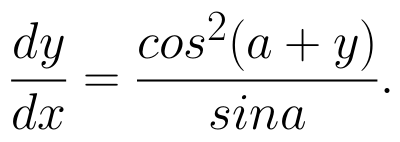Hence show that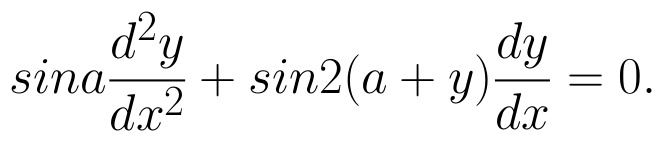(View Answer Video)

Question 3 : Find the general solution of differential equation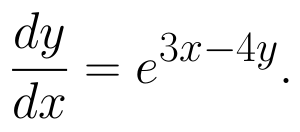(View Answer Video)

Question 4 : Find the general solution of differential equation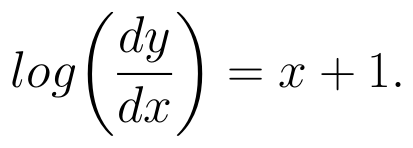(View Answer Video)

Question 5 : Find the particular solution of the differential equation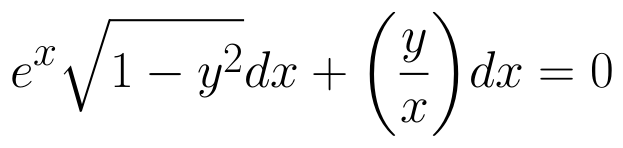given that y = 1 when x = 0. (View Answer Video)

### Matrices

Question 1 : Let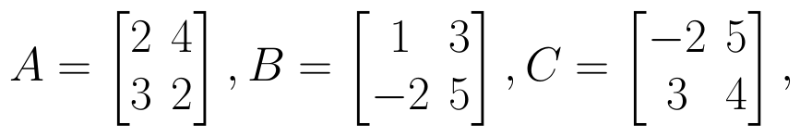Find AB. (View Answer Video)

Question 2 : Find the transpose of the matrix: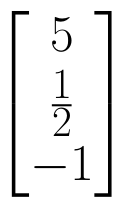. (View Answer Video)

Question 3 : If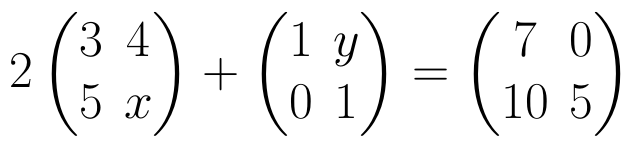, find (x-y). (View Answer Video)

Question 4 : LetFind 3A - C. (View Answer Video)

Question 5 : Matrices A and B will be inverse of each other only if : (View Answer Video)

### Relations and Functions

Question 1 : Let A ={1, 2, 3}. Then number of equivalence relations containing (1, 2) is: (View Answer Video)

Question 2 : A functiondefined as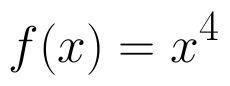is, (View Answer Video)

Question 3 : Let * be the binary operation on N given by a * b = LCM of a and b. Find the identity of * in N? (View Answer Video)

Question 4 : Let * be the binary operation on N given by a * b = LCM of a and b. Find the identity of * in N. (View Answer Video)

Question 5 : Functions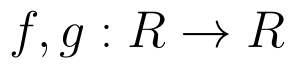are defined respectively, by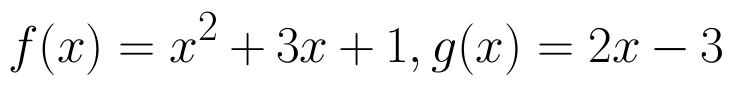, find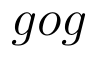(View Answer Video)

### Application of Integrals

Question 1 : Find the area enclosed by the parabola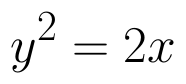and the line x - y = 4. (View Answer Video)

Question 2 : Using integration, find the area of the region bounded by the curves: y = |x + 1| + 1, x = -3, x = 3, y = 0. (View Answer Video)

Question 3 : Find the area of smaller region bounded by the ellipse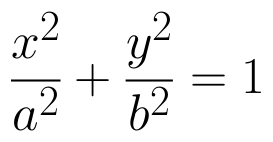and the line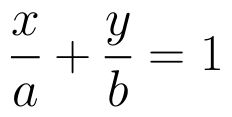. (View Answer Video)

Question 4 : Area lying in the first quadrant and bounded by the circle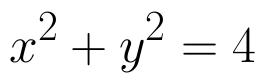and the lines x =0 and x = 2 is (View Answer Video)

Question 5 : Using integration find the area of the triangular region whose sides have the equations y = 2x + 1, y = 3x + 1 and x = 4. (View Answer Video)